output.to from Sideway
Draft for Information Only

# Content

`Magnetic Force in Moving Metal Bar Electricity in Moving Metal Bar Electric and Magnetic Forces Relativity of Electric and Magnetic Fields Source and Reference`

# Magnetic Force in Moving Metal Bar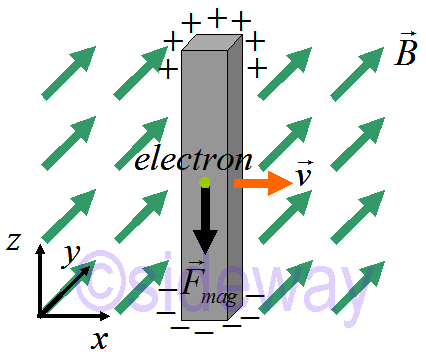By pulling a metal bar through a magnetic field at constant speed 𝑣, electron charges inside the metal bar are moved by magnetic force 𝐹=(-𝑒)𝑣×𝐵 to build up on one side. The electron current flows towards bottom until net force acting on electron is balanced by charge build up, that is 𝐹net=0. ```𝐹net=𝑞𝐸+𝑞𝑣×𝐵=0 ⇒|𝐸|=|𝑣||𝐵| in steady state equilibrium```

## Electricity in Moving Metal Bar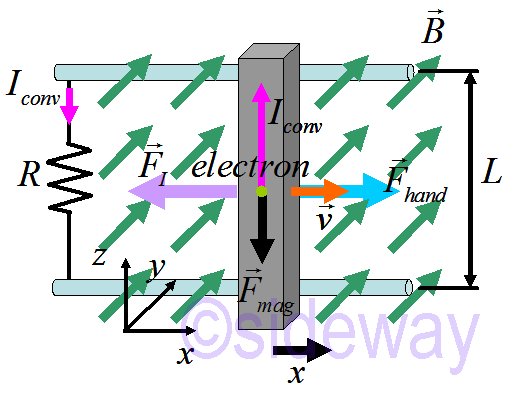By connecting a metal bar to a closed circuit during pulling the metal bar through a magnetic field at constant speed 𝑣, electron charges inside the metal bar are moved by magnetic force along one direction. Since the metal bar is connected to a closed circuit, the current inside the metal bar flows around the closed circuit. The work done by moving the metal bar is ```𝐹𝐼=𝐼∆𝑙×𝐵=−𝐹hand⇒𝐹𝐼=𝐼𝐿𝐵 Work: 𝑊=𝐹∆𝑥=𝐼𝐿𝐵∆𝑥 Power: 𝑃=𝑊∆𝑡=𝐼𝐿𝐵∆𝑥∆𝑡⇒𝑃=𝐼𝐿𝐵𝑣  ∵emf=|𝐸|𝐿=𝑣𝐵𝐿⇒𝑃=𝐼(emf) ```

## Electric and Magnetic Forces

The electric and magnetic forces are 𝐹=𝑞𝐸1=𝑞𝑣×𝐵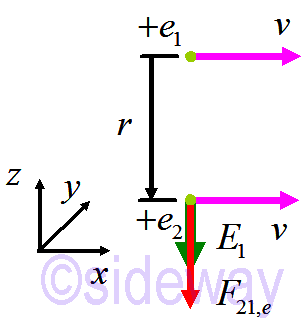The electric field due to 1 on 2 is 𝐸1=14𝜋𝜀0𝑞1𝑟2𝑟
The electric force on 2 due to 1 is 𝐹21,𝑒=𝑞2𝐸1=14𝜋𝜀0𝑞2𝑞1𝑟2𝑟⇒𝐹21,𝑒=14𝜋𝜀0𝑒2𝑟2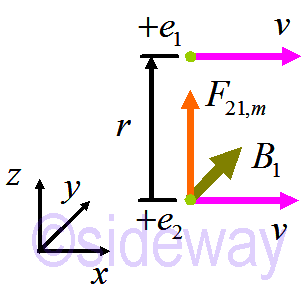The magnetic field due to 1 on 2 is 𝐵1=𝜇04𝜋𝑞1𝑣1𝑟2𝑟
The magnetic force on 2 due to 1 is 𝐹21,𝑚=𝑞2𝑣2×𝐵1=𝑞2𝑣2𝜇04𝜋𝑞1𝑣1𝑟2𝑟⇒𝐹21,𝑚=𝜇04𝜋𝑒2𝑣2𝑟2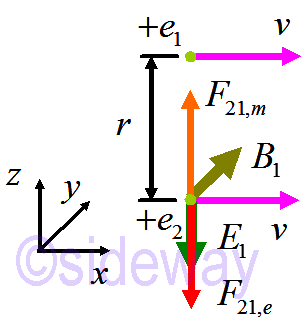The ratio of magnetic force over electric force is 𝐹21,𝑚𝐹21,𝑒=𝜇04𝜋𝑒2𝑣2𝑟2/14𝜋𝜀0𝑒2𝑟2=𝜇0𝜀0𝑣2
Since 𝜇0𝜀0=1𝑐2𝐹21,𝑚𝐹21,𝑒=𝑣2𝑐2
Therefore 𝐹𝑚≤𝐹𝑒

## Relativity of Electric and Magnetic Fields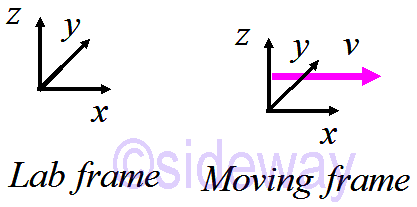Magnetic field measurement depends on frame of reference. The Lorentz factor 𝛾=11−𝑣2/𝑐2>1
Theory of relativity is the speed of light in vacuum = constant 𝑐 = 3×10^8m/s always constant in every references frame. Space and time warp to preserve the speed of light. That is length contraction 𝐿observed=𝐿/𝛾, time dilation 𝑡observed=𝛾*𝑡, mass increase 𝑚observed=𝛾*𝑚. Electric and magnetic field also adjust. Special relativity is wiggy. Since electric and magnetic fields are closely related, they are usually called electromagnetic field.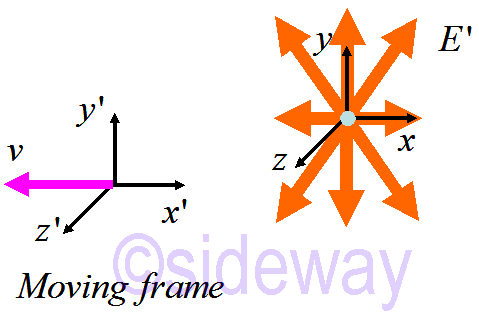The observed electromagnetic field of a rapidly moving particle is ```𝐸′𝑥=𝐸𝑥 𝐸′𝑦=𝛾(𝐸𝑦−𝑣𝐵𝑧) 𝐸′𝑧=𝛾(𝐸𝑧+𝑣𝐵𝑦) 𝐵′𝑥=𝐵𝑥 𝐵′𝑦=𝛾(𝐵𝑦+𝑣𝐸𝑧/𝑐2) 𝐵′𝑧=𝛾(𝐵𝑧−𝑣𝐸𝑦/𝑐2) ```

## Source and Reference

ID: 200101202 Last Updated: 12/1/2020 Revision: 0Home 5

Management

HBR 3

Information

Recreation

Culture

Chinese 1097

English 337

Computer

Hardware 151

Software

Application 198

Manim 121

Numeric 19

Programming

Web 283

Unicode 494

HTML 65

CSS 58

ASP.NET 174

OS 389

Python 19

Knowledge

Mathematics

Algebra 25

Geometry 21

Calculus 67

Engineering

Mechanical

Rigid Bodies

Statics 92

Dynamics 37

Control

Physics

Electric 27